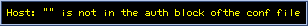Images obtained during our New Zealand Tour
(11-19 Aug. 2001) near the Lake Tekapo6th Oct. 2001: Most of images are renewed

Lake Tekapo and my compact mobile system used in New Zealand

List of 20 images obtained in NZ (Click the names or the small images shown below)
NGC1365, NGC1313, NGC1316, NGC1510&1512, NGC2070 (Tarantula), SN1987a (Super Nova Remnant), NGC5128 (Centaurus A), NGC4945, NGC6164, NGC6300, NGC6302 (Bug Nebula), ESO-138-29, NGC6744, NGC6729 (Valiavle Nebula), NGC6872, IC5152, NGC7410, NGC7793, IC5332NGC55
L=60 min, R=G=5 min (2x2), B=10 min (2x2) with C8 (F=5) + ST-7ETarantula nebula (NGC2070)
L=15 min, R=G=5 min (2x2), B=10 min (2x2) with C8 (F=5) + ST-7ENGC6164
Note that the bright central star of NGC6164 makes the exposure with NABG camera difficult because of blooming. "Non-Blooming Rotational (NBR) composite", developped by us (Motoki, Uto & Okano), has been used here in order to remove the blooming effect.
For more detail on the NBR composite, please see the explanation by Dr. Motoki.
Exposure 1 (in horizontal position): L=20 min, R=G=5 min (2x2), B=10 min (2x2)
Exposure 2 (in vertical position) : L=20 min, R=G=5 min (2x2), B=10 min (2x2)
Two set of the images were combined with "lower value' option.
C8 (F=8.7) + AO-7 + ST-7E
Compare the image without NBR composite.NGC1365 (117kB)
L=25 min, R=G=5 min (2x2 bin), B=10 min (2x2 bin), with C8 (worked at F=5) + ST-7E
Small size (66kB)NGC4945 (128kB)
L=40 min, R=G=5 min, B=10 min with C8 (F=5) + ST-7E
Also note a very faint galaxy PGC45320 (bottom left). North is up.
Small size (83kB)Centaurus A (NGC5128) (75kB)
L=60 min, R=G=B=5 min(2x2 bin) with C8 (F=8.7) + AO-7 + ST-7E
Small size(51kB)Bug Nebula (NGC6302)
L=20 min, R=G=5 min(2x2), B=10min(2x2) with C8 (F=8.7) + AO-7 + ST-7E
NOTE: The visual size of NGC6302 is only 2' x 1', i.e. similar to M57.Variable Nebula (NGC6729)
L=25 min, R=G=5 min(2x2), B=10min (2x2) with C8 (F=8.7) + AO-7 + ST-7ENGC6744
L=60 min, R=5 min(2x2), G=B=10 min (2x2) with C8 (F=6.1) + AO-7 + ST-7ENGC1313
L=20 min, R=G=5 min(2x2), B=10 min(2x2) with C8 (F=5) + ST-7E
NGC1313 is known as a star burst galaxy. It looks like a disturbed SB type(?)NGC7793
L=60 min, R=Y=10 min with C8 (F=8.7) + AO-7 + ST-7ENGC7410
L=30 min, R=5 min (2x2), G=B=10min (2x2) with C8 (F=8.7) + AO-7 + ST-7ENGC6872
L=60 min, R=Y=10 min with C8 (F=8.7) + AO-7 + ST-7ESN1987a in LMC
L=300 min, R=1200 min with C8 (F=8.7) + AO-7 + ST-7E
The bottom RGB image is combined as G=B=(2.56*L - R) in order to extract the red line emission from SN1987a.ESO138-IG29,30
L=5 min (2x2 bin)
The picture of these galaxies by ESO is very fomus.NGC1316 (Type S0?)
L=10 min with C8 (F=5) + ST-7E
Note the dust line on NGC1316. The spiral galaxy (upper) is NGC1317.NGC1510 (Type SB)
L=10 min with C8 (F=5) + ST-7ENGC6300
L=20 min with C8 (F=5) + ST-7EIC5152
L= 20 min with C8 (F=5) + ST-7E
Note: The strong blooming of the brightest star was eliminated by using some filters in PhotoShop. When the blooming line of the bright star is not "on" the target, such cosmetology is easy but it is essentially different from our NBR composite which "restores" the image under the blooming line. The image before this cosmetologyIC5332
L= 20 min with C8 (F=5) + ST-7E

What is LYR?M82 by a LYR Composit with ST-7E

L: 60 min, Y=10 min, R=10 min with C8 + AO-7 + ST-7E
The LYR Composit allows to use the plastic filters (very thin and cutable easily) and to cover only the main CCD of ST-7E without obstructing the light pass to the guide CCD. The calculation is simple; B=L-Y, G=Y-R. The effect on the focus by inserting the thin plastic filters can be neglected in practice, especially for the guide CCD.(in Japanese or in English)

•